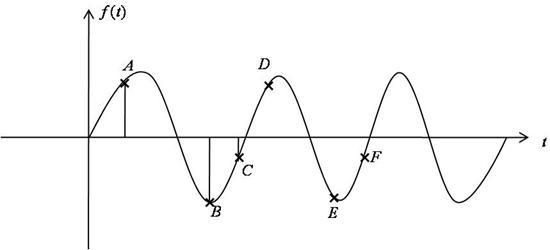# Quiz Chapter 11.04: Discreet Fourier Transform

 MULTIPLE CHOICE TEST DISCREET FOURIER TRANSFORM FAST FOURIER TRANSFORMS

1. Given that $W=e^{-i(2 \pi / N)}$, where $N=3$. Then $F=W^{N}$ can be computed as $F=$

2. Given that $W=e^{-i(2 \pi / N)}$, where $N=3$. Then $F=W^{N/2}$ can be computed as $F=$

3. Given that $N=2$, $\{ f \} = \begin{Bmatrix}4-6i\\-2+4i\end{Bmatrix}$. The values for vector $\{\widetilde{C}^{R}\}$ shown in

• $\widetilde{C}_{n}^{R}=\displaystyle\sum_{k=0}^{N-1}\{f^{R}(k) \cos (\theta)+f^{I}(k) \sin (\theta)\}$

can be computed as:

4. Given that $N=2$, $\{ f \} = \begin{Bmatrix}4-6i\\-2+4i\end{Bmatrix}$. The values for $\{\widetilde{C}^{I}\}$ shown in Equation $(22D)$

• $\widetilde{C}_{n}^{I}=\displaystyle\sum_{k=0}^{N-1}\{f^{I}(k) \cos (\theta)-f^{R}(k) \sin (\theta)\}$

can be computed as:

5. The forcing function $F(t)$ is given as:

• $F(t) = \displaystyle\sum_{n=0}^{7} 10 \sin (2 \pi n t)$

To avoid the aliasing phenomenon, the minimum number of sample data point $N_{min}$ should be

6. Based on the figure belowaliasing phenomena will not occur because there were